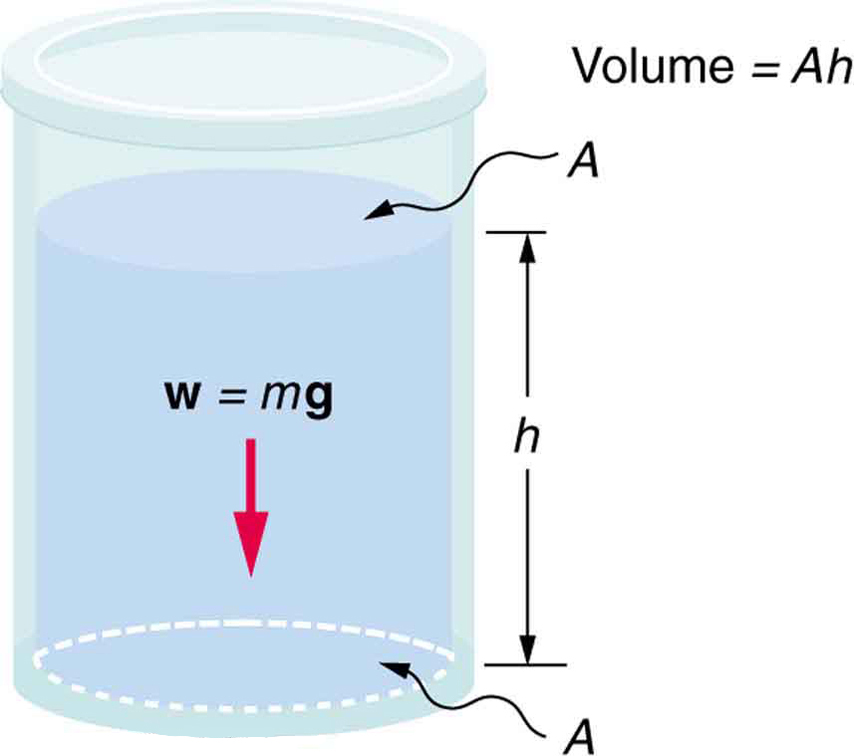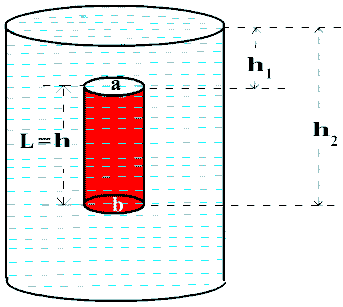# Pressure

Pressure is defined as the force acting per unit area surface.

Pressure = force/ area =F/A……1

S.I unit is Nm-2 is also called 1 Pascal (Pa). Pressure is scalar quantity.

Pressure is inversely proportional to the area. i.e. force acting on the small area exerts more pressure.

Pressure is directly proportional to the applied force.i.e. more pressure is exerted if  the force is increased and vice-versa.

Liquid pressure:

The pressure exerted by the liquid in a vessel is called liquid pressure. Consider a liquid with density d in a beaker of cross sectional area A and height of liquid column is h.P=F/A

P=m g/A

P=d Vg/A

P=d A hg /A

P=d h g……..1

The factors affecting the liquid pressure are:

Density of the liquid

Depth of liquid

Acceleration due to the gravity

Consequences of liquid pressure:

The pressure exerted by a liquid increases with depth.

A liquid finds its own level

The pressure at any point in a liquid acts in all direction.

Pascal’s Law: Pascal’s law of liquid pressure states that, “The pressure is equally exerted perpendicularly on all sides as pressure is applied on a liquid kept in a closed container.”

Instruments based on Pascal’s Law are: Hydraulic Brake and Pistons.

Up thrust: Up thrust is defined as the resultant thrust that a liquid uses to push up a body immersed in the liquid. Its SI unit is Newton.

Density: Density of the substance is defined as the mass per unit volume of that substance.

Density (d) = mass/volume

Density is measured in kg/m.in S.I system. And In c.g.s system it is measured in g/cm3.

Relative density: The ratio of the density of the substance to the density of the substance at 40C is called relative density of the substance. It is unit less.

Archimedes Principle:

It states that,” When a body is partially or wholly immersed in a liquid, it experiences an up thrust equal to the weight of the liquid displaced by it.” This principle is used to calculate up thrust or apparent weight of an object.Let us consider a cylinder of height h and cross-sectional area A completely immersed in a liquid as shown in figure.

Top face be at height of h1 and bottom face be at height h2

Thrust acted on the upper face of the cylinder due to liquid = pressure *area

F1= p1*A

F=dgh1 *A     …………………1

Similarly upthurst acting on the bottom face F2 = p2*A

F2 =dgh2 * A

Total up thrust acting on the body = F2 – F1

U = dgh2 * A – dgh1 *A                                                                                                                  U   = d g A(h2 – h1)

U   = dgV

So the up thrust of the body immersed in a liquid is directly

Density of the liquid

Acceleration due o gravity

Volume of the liquid

Also, the weight of the liquid displaced = dgV, which is equal to the up thrust acting on the body.

Law of flotation:

Law of floatation states that, “The weight of the floating body is equal to the weight of the liquid displaced by the body.”

The density of liquid is directly proportional to the floatation of the object. It means that if the density of liquid is more than the object, then the object floats whereas if the density of liquid is less than that of the object, then the object will sink.  Hydrometer is a device which is based on the principle of flotation and used to measure the specific gravity and density of liquids.